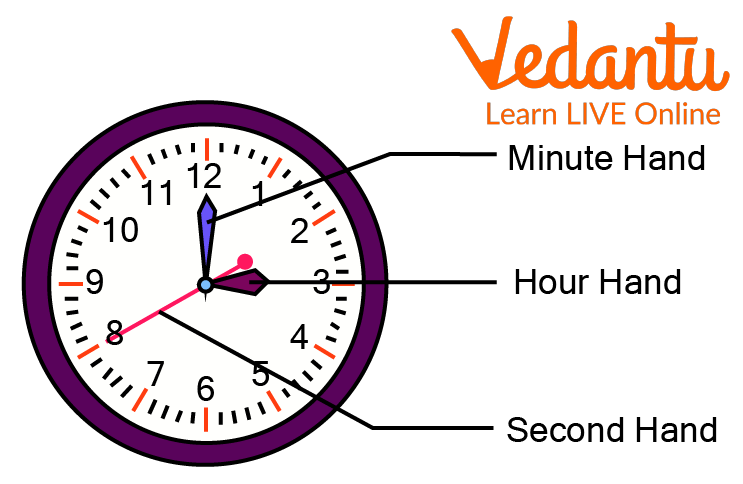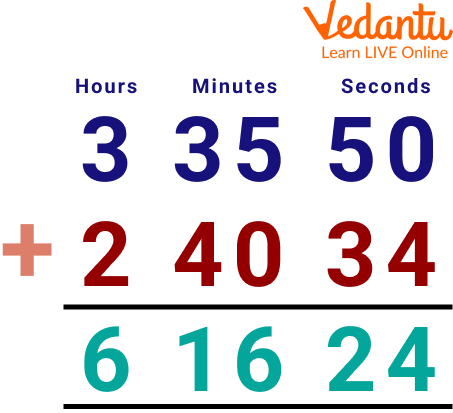Courses
Courses for Kids
Free study material
Offline Centres
More

# Addition of Hours, Minutes, and SecondsLast updated date: 05th Dec 2023
Total views: 117.3k
Views today: 4.17k## A Brief Introduction to the Addition of Hours, Minutes, and Seconds

The addition of time is similar to the addition of numbers, but here, we need to consider the conversion for the addition of time. One unit of time is made up of 60 subunits and is different from the other mathematical conversion units. Here, we will learn how to convert them according to the given problem. For instance, we need to apply this notion of the addition of time to determine the total amount of time spent travelling across various forms of transportation. In this article, we will learn how to add time accurately by using the step-by-step procedure. With the help of some examples, you will learn about conversion and the addition of time.A Clock showing Minutes, Hours, and Second hand

## Time Definition

Time can be defined as the sequence of events that takes place in the present, past, and future, and it is measured using seconds, minutes, hours, days, weeks, months, and years.

• A period of time equal to \$\dfrac{1}{24}\$ of a day is called an hour.

• The S.I. unit of time is a second, and a collection of 60 seconds equals 1 minute.

• A collection of 60 minutes is equal to 1 hour.

## Units of Time

The basic units of time are as follows:

• 1 day = 24 hours

• 1 hour = 60 minutes

• 1 minute = 60 seconds

## Adding Hours, Minutes, and Seconds

By following the simple steps provided below, we can add hours, minutes, and seconds.

2. Now, minutes addition takes place and if the number of minutes is 60 or more, then do the following:

• Subtract 60 from the number of minutes.

• Increase the number of hours by one.

• If the number of minutes is still 60 or more, repeat the same process.

4. When the number of seconds is 60 or more, then do the following:

• Subtract 60 from the number of seconds.

• Increase the number of minutes by one.

• If the number of seconds is still 60 or more, repeat the same process.

Below is an example to understand the above process more clearly.

Add 3 hours 45 minutes 30 seconds and 2 hours 20 minutes 24 seconds.

Step 1: On adding hours together, we get 3 + 2 = 5 hours.

Step 2: Doing minutes addition, we get 45 + 20 = 65 minutes. Since the number of minutes is more than 60, subtract 60 minutes from that, i.e., 65 - 60 = 5 minutes, and increase the number of hours by 1, i.e., 5 + 1 = 6 hours.

Step 3: On adding seconds together, we get 30 + 24 = 54 seconds. So, the number of seconds is less than 60.

Therefore, the result will be 6 hours 5 minutes 54 seconds.

## Solved Examples

1. Compute the addition of time.

(a) 2 hours 25 minutes and 1 hour 15 minutes.

Solution: Adding hours and minutes is done by following the given steps:

Step 1: Add the hours together, we get 2 + 1 = 3 hours

Step 2: Performing minute addition, we get 25 + 15 = 40 minutes

Therefore, the result of the addition will be 3 hours 40 minutes.

(b) 3 hours 35 minutes 50 seconds and 2 hours 40 minutes 34 seconds.

Solution: Adding hours, minutes, and seconds by following the given steps:

Step 1: On adding hours together, we get 3 + 2 = 5 hours

Step 2: Doing minutes addition, we get 35 + 40 = 75 minutes. Since the number of minutes is more than 60, subtract 60 minutes from that, i.e., 15 minutes, and increase the number of hours by 1, i.e., 5 + 1 = 6 hours.

Step 3: On adding seconds together, we get 50 + 34 = 84 seconds. As the number of seconds is more than 60, subtract 60 seconds from that, i.e., 24 seconds, and increase the number of minutes by 1, i.e., 15 + 1 = 16 minutes.

Therefore, the result will be 6 hours 16 minutes 24 seconds.Adding Hours and Minutes along with Seconds

2. Ruth started his homework at 6:30 P.M and completed it at 8 P.M. How many hours and minutes were spent by Ruth to complete her homework?

Solution: We need to subtract 6:30 from 8:00. As we know, 8 hours = 7 hours and 60 minutes. Therefore, (7 hours 60 minutes - 6 hours 30 minutes) equals 1 hour and 30 minutes. Therefore, Ruth spends 1 hour and 30 minutes completing his homework.

## Practice Questions

1. Compute the addition of time 2 hrs 30 min 5 sec and 1 hr 20 min 3 sec.

Ans: 3 hrs 50 mins 8 sec

2. Add 3 hrs 45 min 3 sec and 2 hrs 50 min 4 sec.

Ans: 6 hrs 35 mins 7 sec

3. Anna plans to visit a zoological park. She takes a cab at 3.45 P.M. It takes about 1 hour and 5 minutes to reach her destination. At what time does Anna reach the zoological park?

Ans: 4:50 P.M.

## FAQs on Addition of Hours, Minutes, and Seconds

1. How do you convert minutes into hours?

60 minutes is equal to 1 hour. For converting minutes to hours, divide the number of minutes by 60.

2. How do you write hours and minutes in decimals?

For example, for 56 minutes, we simply divide by 60 minutes: \$\dfrac{56}{60} = .93333333\$. Decimal hours are limited to displaying two decimal places, so the repeating 3 is rounded so that 56 minutes (:56) is expressed in decimal format as .93 hours.

3. How many hours are there in two days?

24 hours is equal to one day, and in two days, we have 2 x 24 = 48 hours. Therefore, the number of hours in 2 days is 48 hours.